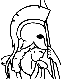#Convex Analysis and Optimization Table of Contents:

1. Basic Convexity Concepts

1. Linear Algebra and Real Analysis
1. Vectors and Matrices
2. Topological Properties
3. Square Matrices
4. Derivatives
2. Convex Sets and Functions
3. Convex and Affine Hulls
4. Relative Interior, Closure, and Continuity
5. Recession Cones
1. Nonemptiness of Intersections of Closed Sets
2. Closedness Under Linear Transformations
6. Notes, Sources, and Exercises

2. Convexity and Optimization

1. Global and Local Minima
2. The Projection Theorem
3. Directions of Recession and Existence of Optimal Solutions
1. Existence of Solutions of Convex Programs
2. Unbounded Optimal Solution Sets
3. Partial Minimization of Convex Functions
4. Hyperplanes
5. An Elementary Form of Duality
1. Nonvertical Hyperplanes
2. Min Common/Max Crossing Duality
6. Saddle Point and Minimax Theory
1. Min Common/Max Crossing Framework for Minimax
2. Minimax Theorems
7. Notes, Sources, and Exercises

3. Polyhedral Convexity

1. Polar Cones
2. Polyhedral Cones and Polyhedral Sets
1. Farkas' Lemma and Minkowski-Weyl Theorem
2. Polyhedral Sets
3. Polyhedral Functions
3. Extreme Points
1. Extreme Points of Polyhedral Sets
4. Polyhedral Aspects of Optimization
1. Linear Programming
2. Integer Programming
5. Polyhedral Aspects of Duality
1. Polyhedral Proper Separation
2. Min Common/Max Crossing Duality
3. Minimax Theory Under Polyhedral Assumptions
4. A Nonlinear Version of Farkas' Lemma
5. Convex Programming
6. Notes, Sources, and Exercises

1. Directional Derivatives
4. Subgradients of Extended Real-Valued Functions
5. Directional Derivative of the Max Function
6. Conical Approximations
7. Optimality Conditions
8. Notes, Sources, and Exercises

5. Lagrange Multipliers

1. Introduction to Lagrange Multipliers
2. Enhanced Fritz John Optimality Conditions
3. Informative Lagrange Multipliers
1. Sensitivity
2. Alternative Lagrange Multipliers
4. Pseudonormality and Constraint Qualifications
5. Exact Penalty Functions
6. Using the Extended Representation
7. Extensions Under Convexity Assumptions
8. Notes, Sources, and Exercises

6. Lagrangian Duality

1. Geometric Multipliers
2. Duality Theory
3. Linear and Quadratic Programming Duality
4. Existence of Geometric Multipliers
1. Convex Cost -- Linear Constraints
2. Convex Cost -- Convex Constraints
5. Strong Duality and the Primal Function
1. Duality Gap and the Primal Function
2. Conditions for No Duality Gap
3. Subgradients of the Primal Function
4. Sensitivity Analysis
6. Fritz John Conditions when there is no Optimal Solution
1. Enhanced Fritz John Conditions
2. Informative Geometric Multipliers
7. Notes, Sources, and Exercises

7. Conjugate Duality

1. Conjugate Functions
2. Fenchel Duality Theorems
1. Connection of Fenchel Duality and Minimax Theory
2. Conic Duality
3. Exact Penalty Functions
4. Notes, Sources, and Exercises

8. Dual Computational Methods